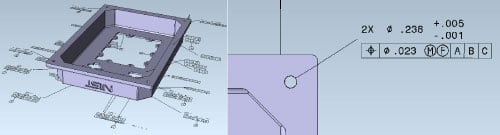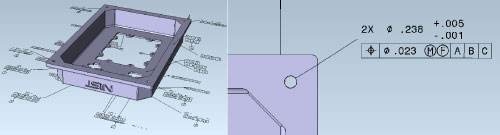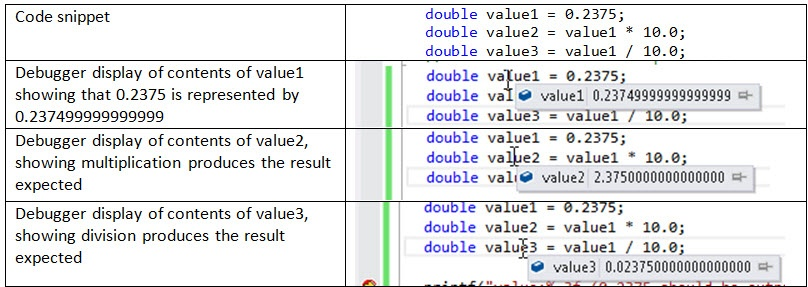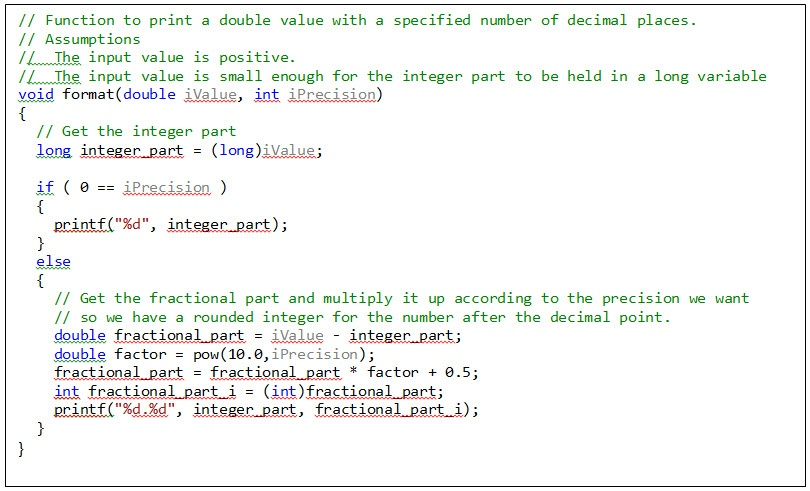# Rounding Dimension Values from 3D InterOp

Wed Aug 16, 2017

Recently I was asked a question about one of the standard test files generated by NIST (National Institute for Standards and Technology) that was being translated by our 3D InterOp translator.  The question concerned the diameter of a hole in the geometry with a dimension on it specifying ⌀0.238, which sounded innocuous enough at first.On closer inspection the dimension in question was showing the size of a hole with a diameter of 0.2375 inches to 3 decimal places, which I found after a bit of searching is the ACME standard drill size for a hole to tap a 90% thread of size 0.3125” with 12 turns per inch.  It would appear to be a perfectly reasonable number but of course there is a gotcha, which is why I am writing this.

My test application printed out the nominal value of the dimension of the hole as 0.237500, the value I expected, however on examining the variable holding that value in the debugger I found it to be 0.237499999999999.  So my initial thought was that someone had done a floating point calculation somewhere and introduced an inaccuracy.

One of the first things that a new developer in geometric modeling gets to learn is that calculations using floating point values can introduce tiny inaccuracies due to the precision of the floating point representation.  Many times I have heard developers discussing how code is behaving differently on two separate platforms because of a difference between two floating point values in the 14th place of decimals.  So I was half right in my guess about where this error came from, but this was not the result of a series of calculations.

In fact, due to the way floating point numbers are represented in computers 0.2375 does not have that exact value when assigned to a double in C++.  I can write some code to assign 0.2375 to a variable and when I look at it in the debugger it is represented as 0.237499999999999.  From the programming point of view it behaves nicely, in that multiplying by 10.0 yields 2.375 and dividing by 10 yields 0.02375.In my test code I was simply using printf to output the nominal value, which looked fine because printf was printing out 6 decimal places giving 0.237500.  If we go back to the original question about a display of ⌀0.238 we can see that the dimension is set up to display with 3 decimal places of precision, and unfortunately that is exactly the precision that will get rounded down due to the inaccuracy of the floating point representation, any other precision would work as expected.

The way to generate the required output string is to treat the number we are trying to format in two parts, to the left and the right of the decimal point.  The left part is obtained by extracting the integer part of the floating point number, assuming the number is not too big to be held in an integer, which for a dimension is reasonable.  The right part is obtained by subtracting the integer part, then multiplying by 10.0 to the power of the number of decimal places, adding 0.5 then taking the integer part of the result.The combination of having a number that is not represented exactly and a display precision such that it gets rounded down means that the chances of this situation occurring are pretty small.  I suspect this is not a coincidence, it is a good test case and I have to wonder if someone at NIST will be chuckling as they read this.

## You May Also Like

These Stories on 3D InterOp